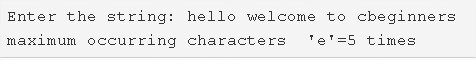# Highest Frequency Character In A String C Program | 4 Ways

C program to find the highest frequency character in a string – In this article, we will detail in on the multiple means to find the highest frequency character in a string in C programming.

Suitable examples and sample programs have also been added so that you can understand the whole thing very clearly. The compiler has also been added with which you can execute it yourself.

The means used in this distinct piece are as follows:

• Using Standard Method
• Using Function

A string is nothing but an array of characters. The value of a string is determined by the terminating character. Its value is considered to be 0.As you can see in the image uploaded above, firstly, you need to enter the string successfully.

The string entered here is as follows:

“hello welcome to cbeginners”

As you can see, there are quite a few characters which are repeated but the character ‘e’ is repeated the most.

It is used for 5 times.

Thus, the maximum frequency is of ‘e’: 5 times.

Hence, doing the same in C programming is as follows:

## Using Standard Method

1. Initialize count=0,k=0.Read the entered string and store the string into the variable s using gets(s).

2) The for loop iterates the string until the last character of the string becomes to null. Then initialize the j value to n, which is the length of the string.

3) a) Iterate the outer for loop through the string with the structure for(i=0;i<n;i++).

b) Inner for loop compares the remaining characters of the string with s[i]. If it matches then increase the count value by 1.

c) Place each character frequency in the integer array a.

d) Initialize k with maximum frequency.

e) If any frequency value of a match with the k then print that element and k value.

Output:

### Using Function

1. The function stringlength(char *s) returns the length of the string.

2) The main() function calls the printmax(char *s) by passing the string as an argument to the function.

3) The function will print the highest frequency elements.

a) The outer for loop iterates through the string, the inner loop finds the frequency of each character and store the frequencies in the string a.

b) initialize the k with maximum frequency.

4) If the frequency of any character is equal to the k value then print that character and k value.

Output:
x

## C Program To Print Number Of Days In A Month | Java Tutoring

C program to input the month number and print the number of days in that ...